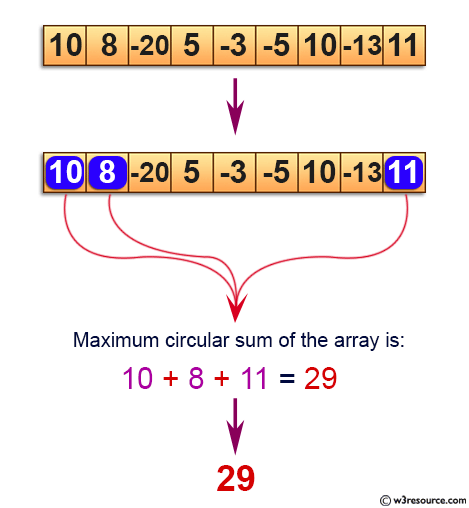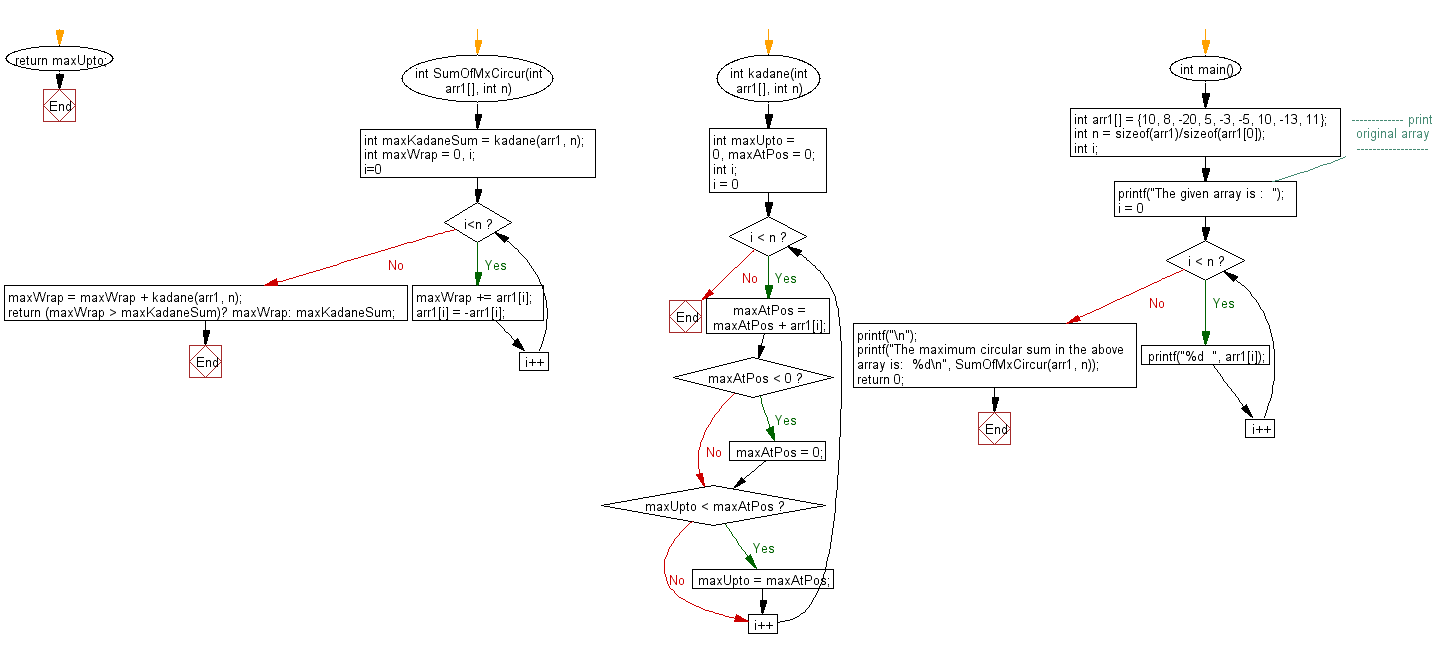﻿ C exercises: Find the maximum circular subarray sum of a given array - w3resource# C Exercises: Find the maximum circular subarray sum of a given array

## C Array: Exercise-51 with Solution

Write a program in C to find the maximum circular subarray sum of a given array.

Pictorial Presentation:Sample Solution:

C Code:

``````#include <stdio.h>

int SumOfMxCircur(int arr1[], int n)
{
int maxWrap = 0, i;
for (i=0; i<n; i++)
{
maxWrap += arr1[i];
arr1[i] = -arr1[i];
}
maxWrap = maxWrap + kadane(arr1, n);
}
{
int maxUpto  = 0, maxAtPos = 0;
int i;
for (i = 0; i < n; i++)
{
maxAtPos = maxAtPos + arr1[i];
if (maxAtPos < 0)
maxAtPos = 0;
if (maxUpto < maxAtPos)
maxUpto = maxAtPos;
}
return maxUpto;
}

int main()
{
int arr1[] =  {10, 8, -20, 5, -3, -5, 10, -13, 11};

int n = sizeof(arr1)/sizeof(arr1);
int i;
//------------- print original array ------------------
printf("The given array is :  ");
for(i = 0; i < n; i++)
{
printf("%d  ", arr1[i]);
}
printf("\n");
//------------------------------------------------------
printf("The maximum circular sum in the above array is:  %d\n", SumOfMxCircur(arr1, n));
return 0;
}```
```

Sample Output:

```The given array is :  10  8  -20  5  -3  -5  10  -13  11
The maximum circular sum in the above array is:  29
```

Flowchart:C Programming Code Editor:

Improve this sample solution and post your code through Disqus.

What is the difficulty level of this exercise?

﻿

## C Programming: Tips of the Day

Where is the C auto keyword used?

auto is a modifier like static. It defines the storage class of a variable. However, since the default for local variables is auto, you don't normally need to manually specify it.

Ref : https://bit.ly/3yzwC9r